# New York is roughly 3000 miles from Seattle. When it is 10:00 A.M. in Seattle, it is 1:00 P.M. in New York

New York is roughly 3000 miles from Seattle. When it is 10:00 A.M. in Seattle, it is 1:00 P.M. in New York. Using this information, estimate (a) the rotational speed of the surface of Earth, (b) the circumference of Earth, and © the radius of Earth.

The distance d travelled by an object is equal to the product of the speed v of the object and
the time taken t by it.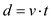(a)
Calculate the rotational speed of the surface of Earth as follows:Solve for v by substituting 3000 miles for d and 3 hours for t.Therefore, the rotational speed of the surface of Earth is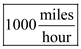(b)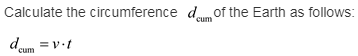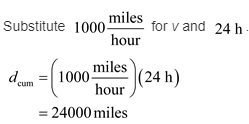Therefore, the circumference of the Earth is 24000 miles .

( c)
Calculate the radius of the Earth as follows: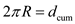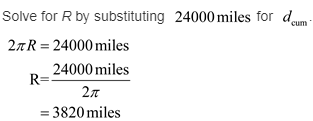Therefore, the radius of the Earth is 3820 miles .